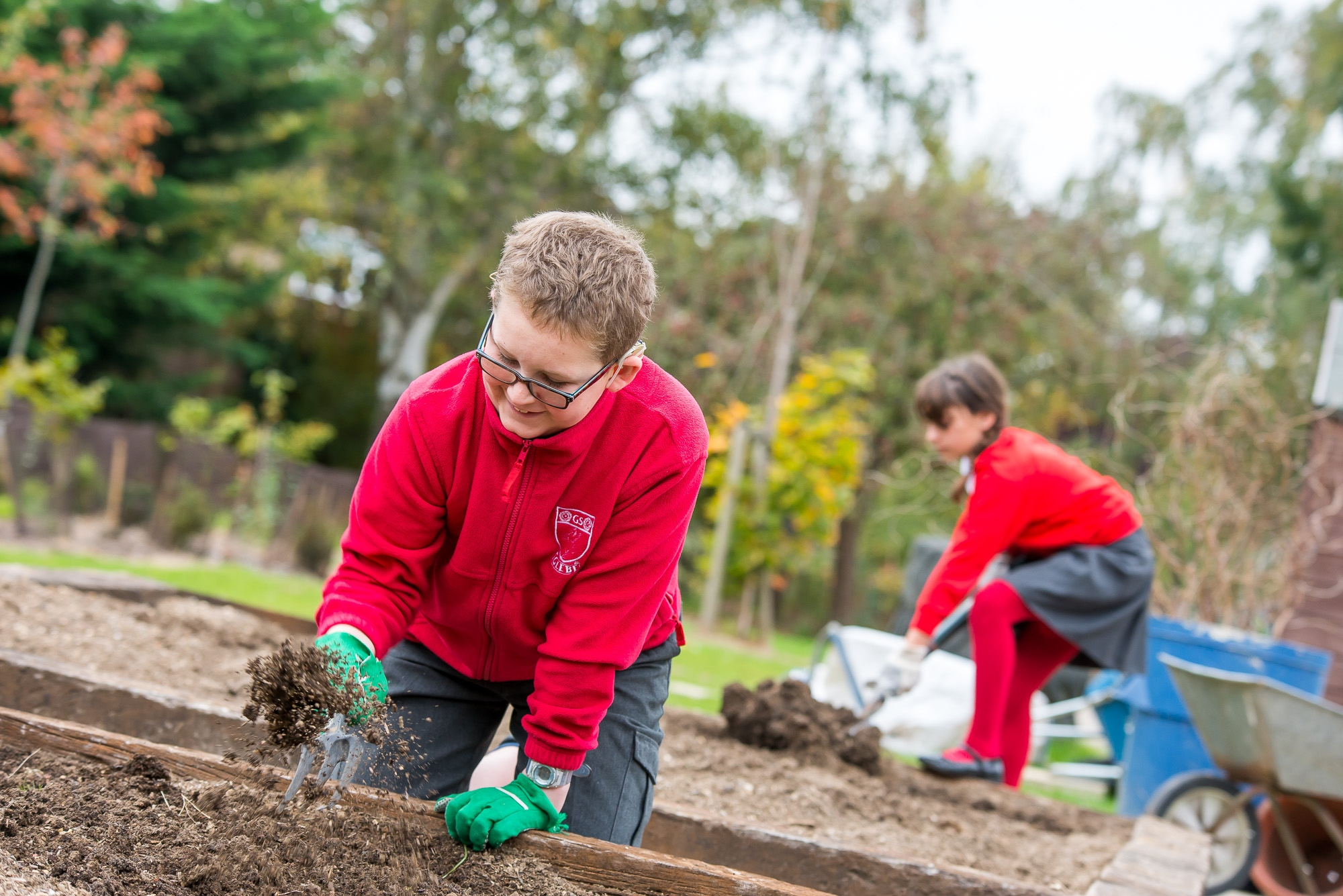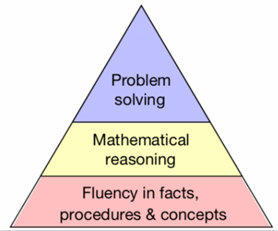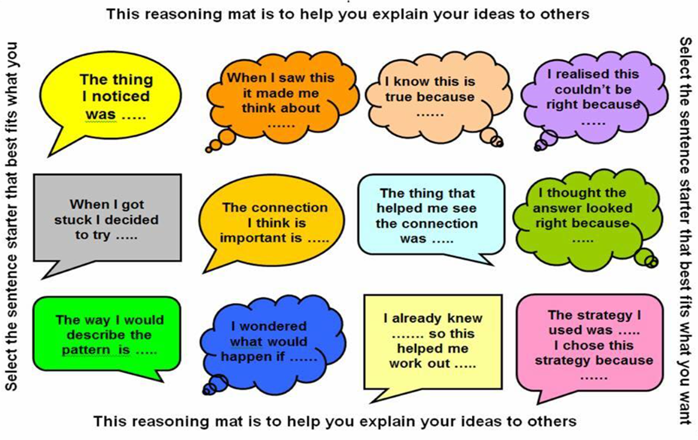# ReasoningAt Glebe, we place high importance in the development of our pupils’ mathematical reasoning. On a day-to-day basis, our maths lessons comprise of a combination of fluency, reasoning and problem solving. Becoming fluent in maths (knowing procedures and facts) goes hand-in-hand with reasoning to ensure children become confident problem-solvers.

## What is reasoning?

Reasoning in maths is the process of applying logical thinking to a situation to derive the correct problem solving strategy for a given question, and using this method to develop and describe a solution. Put more simply, mathematical reasoning is the bridge between fluency and problem solving. It allows pupils to use the former to accurately carry out the latter, and encourages the pupil to think about the ‘why?’.

## Why is reasoning important?

Research by Nunes (2009) states that ‘ability to reason mathematically is the most important factor in pupil’s success in mathematics. Such skills support deep and sustainable learning and enable pupils to make mathematical connections’.

Reasoning about what is already known in order to work out what is unknown will improve fluency; for example, if I know what 12 × 12 is, I can apply reasoning to work out 12 × 13. The ability to reason also supports the application of mathematics and an ability to solve problems set in unfamiliar contexts.

How can you support with reasoning at home?

• Talk about maths in a positive way. A positive attitude about maths is infectious.
• Encourage independence. If children are stuck with their maths homework don't step in straight away. Step back and give your child time to think independently about which strategies they could apply.
• Encourage persistence. Some problems take time to solve.
• Encourage your child to experiment with different approaches to mathematics. There is often more than one way to solve a maths problem.
• Encourage your child to talk about their maths and explain how they came to their answer. When your child is solving math problems ask questions such as: Why did you...?  What can you do next? Do you see any patterns?  Does the answer make sense? How do you know? This helps to encourage thinking about mathematics. (Use the question mat below to help with this).
•• Connect maths to everyday life and help your child understand how math influences them (i.e. shapes of traffic signs, walking distance to school, telling time).